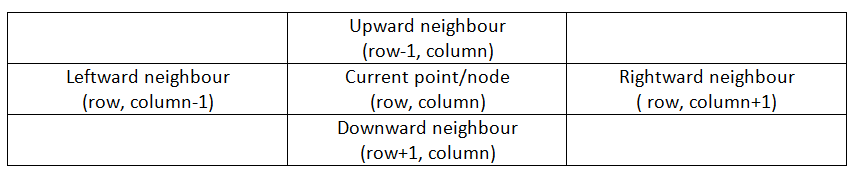Q:

# Shortest Source to Destination Path

Given a Boolean 2D matrix (0-based index), find whether there is a path from (0,0) to (x,y) and if there is one path, print the minimum no of steps needed to reach it, else print -1 if the destination is not reachable. Moves are possible in only four directions i.e. up, down, left and right. The path can only be created out of a cell if its value is 1.

Example:

```    Matrix dimension: 3X3
Matrix:
1 0 0
1 1 0
0 1 1
Destination point: (2, 2)
Shortest path length to reach destination: 4```

Pre-requisites:

1. Defining a point in the maze

We need to define a "point" class having two data attributes 1) row no and 2) column no

```class point{
public:
int row;
int column;
};
```

2. Defining node used in solution

A concept of node is used in the solution which actually is an object with two data attributes

1. A point
2. Distance of the point from the source, distance is calculated by path traversed
```class node{
public:
point p;
int d;
};
```

Algorithm:

1. Start from the source node. (point (0,0) with a distance 0 )
2. Declare a queue for BFS traversal.
3. Check whether a path from the current node is possible or not.
4. If possible
Mark the node visited.
EnQueue its neighbour nodes if unvisited
5. Check for final node to be reached.
6. In case of traversing to the neighbour nodes increment node data distance by 1.
7. Return the final node distance value when final node is reached.
8. If all nodes are processed to make the queue empty, then it isn't possible to be reached
Print -1.

Since we have use BFS traversal technique it's guaranteed to reach the destination node in minimum no of steps if the destination is reachable from the source node. (point (0, 0)).

So the steps are:

1. Checking the base cases
Check whether point (0,0) is 0 or not.
If it's 0, then we can't make any path from here, so to print -1 & return.
2. Initialize Mark[row][col] = false
Initially all nodes are unvisited
3. Initialize the queue q
4. Create source node & EnQueue(q, source node).
5. Start traversal
```While (queue is not empty)
Temp=DeQueue(q)
IF temp==destination node
printnode distanceand return
END IF
For all neighbour nodes //increment distance by 1
Check whether valid node && unvisited
IFit's a valid node && unvisited
EnQueue(neighbour node, q)
Mark neighbour node visited
END IF
END FOR
END WHILE
```
1. If control comes out of the loop that means destination can't be reached, thus print -1.

Finding neighbour nodesAll the directions are marked on basis of the current index.

• For rightward neighbour-> (row, column+1)
• For downward neighbour-> (row+1, column)
• For leftward neighbour -> (row, column-1)
• For upward neighbour-> (row-1, column)

Checking valid node

```If (row of current point<0)
Out of matrix;
If (column of current point<0)
Out of matrix;
If (row of current point>=m) //m is row no of matrix
Out of matrix;
If (column of current point>=n) //n is column no of matrix
Out of matrix;
```

### C++ implementation

``````#include <bits/stdc++.h>
using namespace std;

//checking valid node
int isvalid(int nextx,int nexty,int m,int n){
if(nextx>=0 && nextx<m && nexty>=0 && nexty<n)
return 1;
return 0;
}

//defining point
class point{
public:
int row;
int col;
//make point
void mpoint(int m,int n){
row=m;
col=n;
}
};

//defining node
class node{
public:
point p;
int d;
void mnode(point q,int dis){ //make node
p.row=q.row;
p.col=q.col;
d=dis;
}
};

//finding shortest path
int shortest(int** a,int m,int n,int x1,int y1){
if(a==0)//base case
return -1;

bool visited[m][n];
//initialize
memset(visited,false,sizeof(visited));

//declare queue by STL
queue<node> q;

point curr;
//set the source point (0,0)
curr.mpoint(0,0);

node t;
//set the source node at point (0,0)
t.mnode(curr,0);

//ENQUEUE source node
q.push(t);

//direction matrices
int arrx[]={-1,0,1,0};
int arry[]={0,1,0,-1};

point c;
node v;
while(!q.empty()){
//DEQUEUE
v=q.front();

c=v.p;
//if destnation node is reached
if(x1==c.row && y1==c.col ){
return v.d;
}
q.pop();
//check for all four neighbours
for(int i=0;i<4;i++){
int nextx=c.row+arrx[i];
int nexty=c.col+arry[i];
//if valid node && not visited yet
if(isvalid(nextx,nexty,m,n) && a[nextx][nexty] && !visited[nextx][nexty]){
curr.mpoint(nextx,nexty);
//set neighbour node by incrementing distance value
t.mnode(curr,(v.d)+1);
q.push(t); //EnQueue
//mark as visited
visited[nextx][nexty]=true;
}
}
}
return -1;
}

int main()
{
int m,n,x,y;

cout<<"enter matrix row & column"<<endl;
scanf("%d %d",&m,&n);

int **a=(int**)malloc(sizeof(int*)*m);

for(int i=0;i<m;i++)
a[i]=(int*)(malloc(sizeof(int)*n));

cout<<"enter matrix elements (0/1)"<<endl;
for(int i=0;i<m;i++)
for(int j=0;j<n;j++)
scanf("%d",&a[i][j]);

cout<<"enter row & column of destinanation point"<<endl;
scanf("%d %d",&x,&y);

if(shortest(a,m,n,x,y)!=-1)//if path found
printf("shortest distance is %d\n",shortest(a,m,n,x,y));
else{
cout<<-1<<endl;
cout<<"no path found\n";
}

return 0;
}``````

Output

```First run:
enter matrix row & column
3 3
enter matrix elements (0/1)
1 0 0
1 1 0
0 1 1
enter row & column of destinanation point
2 2
shortest distance is 4

Second run:
enter matrix row & column
4 4
enter matrix elements (0/1)
1 0 1 0
0 0 0 1
1 1 1 1
0 1 1 0
enter row & column of destinanation point
2 3
-1
no path found```